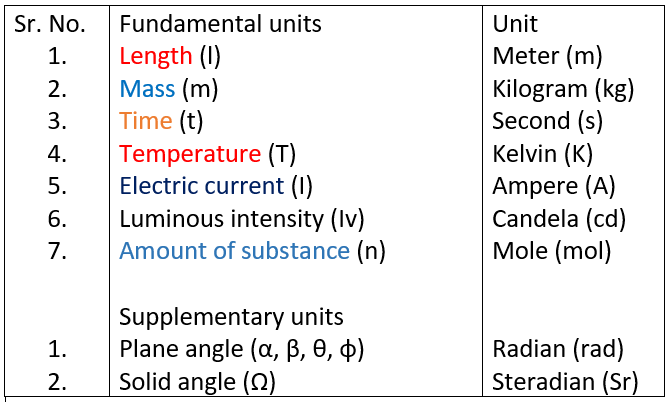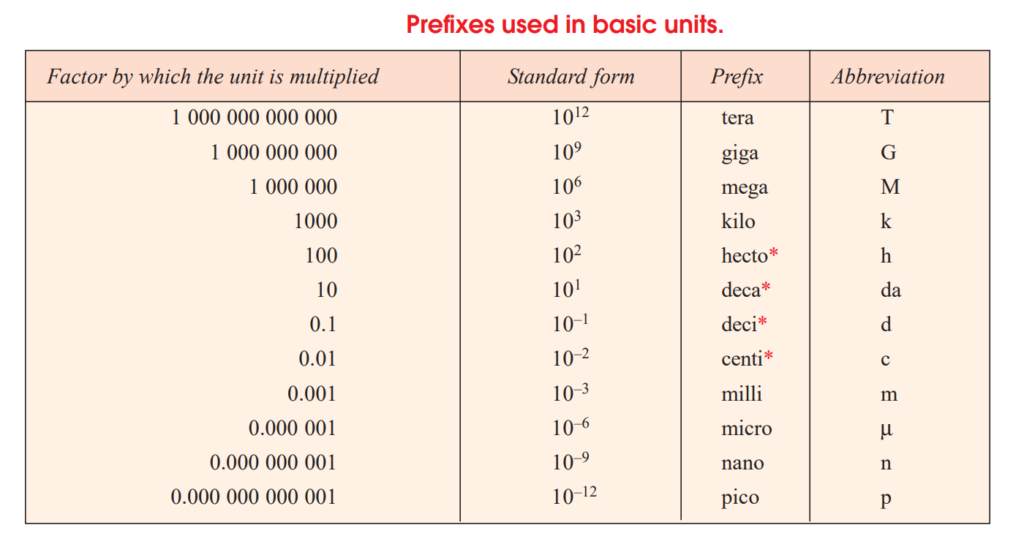October 25, 2021# How to do unit conversions of length-area-weight-pressure

Unit conversions is the big concern of all science and engineering student. We have to use different units in day to day life.in this article we will going to learn how to convert different units of length, weight, area and some engineering unit conversions.

Fundamental Units
The measurement of physical quantities is one of the most important operations in engineering.
Every quantity is measured in terms of some arbitrary, but internationally accepted units, called
fundamental units.
Derived Units
Some units are expressed in terms of other units, which are derived from fundamental units, are
known as derived units e.g. the unit of area, velocity, acceleration, pressure, etc.
System of Units
There are only four systems of units, which are commonly used and universally recognized.
These are known as :
1. C.G.S. units, 2. F.P.S. units, 3. M.K.S. units, and 4. S.I. units.
Since the present course of studies are conducted in S.I. system of units, therefore, we shall
discuss this system of unit only.

## S.I. Units (International System of Units)

In this system of units, there are seven fundamental units and two supplementary units, which
cover the entire field of science and engineering.7 Fundamental and supplementary units.Prefixes used in basic units conversions

For unit conversions you need to know some basic units.
1. Length
C.G.S. : centimeter
M.K.S. : meter
F.P.S. : foot
2. Mass
C.G.S. : gram
M.K.S. : kilogram
F.P.S. : pound
3. Time
C.G.S. : second
M.K.S. : second
F.P.S. : second
4. Current
S.I. : ampere (A)
5. Temperature
S.I. : kelvin (K)
6. Area
C.G.S. : cm2
M.K.S. : m2
7. Volume
C.G.S. : cm3
or cc.
M.K.S. : meter 3
8. Density
C.G.S. : gm/cm3
M.K.S. : kg/meter 3
9. Speed
C.G.S. : cm/sec
M.K.S. : meter/sec
10. Velocity
C.G.S. : cm/sec
M.K.S. : meter/sec
S.I. : km/sec
11. Momentum
C.G.S. : gm cm/sec
M.K.S. : kg.m/sec
12. Force
C.G.S. : dyne
M.K.S. or SI unit: newton (N)
13. Thrust
C.G.S. : dyne or gm wt
M.K.S. : newton or kg.wt
14. Pressure
C.G.S. : dyne/cm2
or g.wt/cm2
M.K.S. : newton/m2
or kg.wt/m2
M.K.S. or SI System: km/pascal
15. Work
C.G.S. : erg or dyne cm
M.K.S. : joule or Nm
16. Power
C.G.S. : erg/sec
M.K.S. : watt
17. Energy
C.G.S. : erg
M.K.S. : joule
18. Acceleration due to gravity (g)
M.K.S. : meter/sec2
19. Moment of Force
C.G.S. unit: dyne cm
S.I. unit : newton metre (Nm)
20. Density
C.G.S. : grams/cm3
M.K.S. : kilogram/m3
21. Heat
C.G.S. : calorie
S.I. : joule (J)
22. Specific heat
C.G.S. : cal/gm°C
M.K.S. : J/kg°C
23. Calorific Value of Specific energy
C.G.S. : cal/gm
M.K.S. : J/kg
22. Frequency
hertz or Cycles/sec
23. Charge
coulombs (C)
24. Capacity
25. Potential
volts (V)
27. Universal Gravitational
Constant (G)
Nm2
/kg2
28. Angular Displacement
29. Angular Velocity
31. Solid angle
32. Luminous Intensity
Candela(cd) or Lumens
33. Candle Power
Candela(cd)
35. Magnetic Induction or Magnetic Flux Density
newton/ampere-meter
36.Intensity of Magnetic field
C.G.S. : Gauss
S.I. : Wb/m2
or tesla
37. Electric Power
joule/sec or watt
38. Resistance
Ohm
39. Specific Resistance
Ohm-meter
40. Quantity of heat
calories

## 1.Length unit conversions

SI unit of length is meter

1 meter = 1000 mm and 1mm= 0.001 meter

= 100 cm and 1cm = 0.01 meter

= 10 decimeter and 1decimeter = 0.1 meter

= 39.37 inch and 1inch = 0.0254 meter

= 3.280 foot and 1foot = 0.3048 meter

= 10^6 micrometer and 1 micrometer = 10 ^-6 meter

= 10^9 nanometer and nanometer = 10 ^-9 meter

= 1.093613 yards and 1 yard = 0.9144 meter

1 kilometer = 1000 meter, 1meter = 0.001 kilometer

= 10000 decimeter, 1 decimeter = 0.001 kilometer

= 1093.6 yard, 1 yard = 0.0009144 kilometer

= 3280.839895 foot, 1 foot = 0.0003048 kilometer

= 39370.07874 inch, 1 inch = 0.0000254 kilometer

=0.621371 miles , 1 mile = 1.60934 kilometer

You will get online length unit conversions.

= 1000 gram

=10^6 milligram

=2.20462 pounds

=35.274 ounce

=0.001 tonne

= 1000 kilogram

=10^6 grams

=10^9 milligram

= 2204.62 pounds

=35274 ounce

## 1 Hectare

= 2.47105 acre

=0.01 square kilometer

=10000 square meter

=0.003861 square mile

=11959.9 square yard

=107639 square foot

=1.55 x 10^6 square inch

## 1 square foot

= 0.090290 square meter

=0.111111 square yard

=144 square inch

## 1 square inch

=0.00694444 square foot

=2.491e-10 square mile

=0.000645166064504 square meter

=1.5942e-7 Acre

## 4. Power unit conversions

• 1 Horsepower (electric) = 746 Watt
• Btu (th)/ minute = 17.5725 watt
• ton (refrigeration) = 3516.85 watt
• calorie (th)/  = 4.184 watt
• joule/minute(J/min) = 0.016666 watt
• pound-foot/ second = 1.3558 watt#### SKY HIGH eLearn

View all posts by SKY HIGH eLearn →